# Shakespeare Is More Monkey-Friendly Than Cervantes

Ford, there is an infinite number of monkeys outside who want to talk to us about this script for Hamlet they have worked out (from Episode 2 of The Hitchhiker’s Guide to the Galaxy by Douglas Adams)

Some days ago I was talking with a friend about the infinite monkey theorem which is a funny interpretation of what thinking-in-infinite can produce. The same day, in my weekly English class, my teacher said that Anglo-saxon words do tend to be short, very often monosyllabic such as function words such as to, of, from etc and everyday words such as go, see run, eat, etc.

Both things made me think that a monkey could have easier to type a Shakespeare text rather than a Cervantes one. I cannot imagine a definitive way to demonstrate this but this experiment support my hypothesis. After simulating random words of 2, 3, 4 and 5 characters I look for them in English(1) and Spanish(2) dictionaries, which I previously downloaded from here. Result: I find more random words in the English one. These are the results of my experiment:For example, around 38% of two-chars words match with English dictionary and only 9% with Spanish one. This is why I think that, in the infinite, I would be easier for a monkey to replicate a Shakespeare text than a Cervantes one.

Here you have the code:

```library(ggplot2)
library(scales)
df.lang=do.call("rbind", list(esp.dic, eng.dic))
df.lang\$WORD=tolower(iconv(df.lang\$WORD, to="ASCII//TRANSLIT"))
df.lang=unique(df.lang)
results=data.frame(LANG=character(0), OCCURRENCES=numeric(0), SIZE=numeric(0), LENGTH=numeric(0))
for (i in 2:5)
{
df.monkey=data.frame(WORD=replicate(20000, paste(sample(c(letters), i, replace = TRUE), collapse='')))
results=rbind(results, data.frame(setNames(aggregate(WORD ~ ., data = merge(df.lang, df.monkey, by="WORD"), FUN=length), c("LANG","OCCURRENCES")), SIZE=20000, LENGTH=i))
}
opt=theme(panel.background = element_rect(fill="gray92"),
panel.grid.minor = element_blank(),
panel.grid.major.x = element_blank(),
panel.grid.major.y = element_line(color="white", size=1.5),
plot.title = element_text(size = 35),
axis.title = element_text(size = 20, color="gray35"),
axis.text = element_text(size=16),
axis.ticks = element_blank(),
axis.line = element_line(colour = "white"))
ggplot(data=results, aes(x=LENGTH, y=OCCURRENCES/SIZE, colour=LANG))+
geom_line(size = 2)+
scale_colour_discrete(guide = FALSE) +
geom_point(aes(fill=LANG),size=10, colour="gray92",pch=21)+
scale_x_continuous("word length", labels=c("two chars", "three chars", "four chars", "five chars"))+
scale_y_continuous("probability of existence", limits=c(0, 0.4), labels = percent)+
labs(title = "What if you put a monkey in front of a typewriter?")+
opt + scale_fill_discrete(name="Dictionary", breaks=c("ESP", "ENG"), labels=c("Spanish", "English"))
```

(1) The English dictionary was originally compiled from public domain sources
for the amSpell spell-checker by Erik Frambach e-mail: e.h.m.frambach@eco.rug.nl
(2) The Spanish dictionary has been elaborated by Juan L. Varona, Dpto. de Matematicas y Computacion, Universidad de La Rioja, Calle Luis de Ulloa s/n, 26004 SPAIN e-mail: jvarona@siur.unirioja.es

# The mnemoneitoR

AND I HAVE A GREAT REJOICING DAY (mnemonic rule generated by mnemoneitoR for first 7 digits of Pi according to The Wonderful Wizard Of Oz)

Is there some number impossible to memorize? Do not worry, here comes mnemoneitoR: the tool that you was always looking for! With mnemoneitoR you can translate any number into an easy-to-remember phrase inspired by your favorite book. It is very easy: choose a book, enter the number and mnemoneitoR will show you as many possibilities as you want. Just choose the one you like most!

There are many webs about mnemonics in the Internet, like this one. One of my favourite menmonic devices for Pi is:

HOW I WANT A DRINK, ALCOHOLIC OF COURSE, AFTER THE HEAVY LECTURES INVOLVING QUANTUM MECHANICS

The number of letters in each word gives the respective number in the sequence (i.e., 3.14159265358979).

For professional purposes, I am learning how to manage texts in R and I discovered a very useful package called `stringr`. This is the only one I need for this experiment. The process is simple: I download a book from Project Gutenberg site, clean and split the text and do simulations on the fly of a Markov Chain generated from the words of the book. Step by step:

• Downloading the book is quite simple. You search the one you want, copy the url in the code (after line “CHOOSE YOUR FAVORITE BOOK HERE”) and no more.
• After loading the text, some easy tasks are needed: remove header and footer lines, split text into words, turn them into uppercase, remove non-text characters … typical things working with texts.
• After reading the number you want to translate, I choose a word sampling along all words with the same number of letters as the first digit with probability equal to the number of appearances. This is how I initialize the phrase. Next word are chose among the set of words which are preceded by the first one and have the same number of letters as the second digit with probability equal to number of appearances, and so on. This is a simulation on the fly of Markov Chain because I do not have to calculate the chain explicitly.
• I always translate Zero with the same word you choose. I like using “OZ” instead Zero.

Most of the phrases do not have any sense but are quite funny. Few of them have some sense and maybe with a small tweak, can change into full of meaning sentences. Here you have some samples of the output of mnemoneitoR:I like how the phrases smell like the original book. I will try to improve mnemoneitoR in the future but I can imagine some uses of this current version: message generator for fortune cookies,  a cool way to translate your telephone number into a sentence …

Here you have the code. If you discover nice outputs in your experiments, please let me know:

```library(stringr)
# CHOOSE YOUR FAVORITE BOOK HERE (Currently "The Wonderful Wizard of Oz")
TEXTFILE = "data/pg55.txt"
# Remove header and footer, concatenate all of the lines, remove non-text and double spaces chars and to upper
textfile = textfile[(grep('START OF THIS PROJECT', textfile, value=FALSE)+1:grep('END OF THIS PROJECT', textfile, value=FALSE)-1)]
textfile <- paste(textfile, collapse = " ")
textfile <- gsub("[^a-zA-Z ]","", textfile)
textfile <- toupper(textfile)
textfile <- gsub("^ *|(?<= ) | *\$", "", textfile, perl=T)
# Split file into words
textfile.words <- strsplit(textfile," ")
textfile.words.freq <- as.data.frame(table(textfile.words));
names(textfile.words.freq) <- c("word", "freq")
textfile.words.freq\$length <- apply(data.frame(textfile.words.freq[,c("word")]), 1, function(x) nchar(x))
number <- 3.1415926
number <- gsub("[^0-9]","", as.character(number))
# Define the word representing Zero
zero.word = "OZ"
fg <- as.integer(substr(number, 1, 1))
df <- textfile.words.freq[textfile.words.freq\$length==fg,]
wd <- sample(df\$word, size=1, prob=df\$freq)
phrase <- c(as.character(wd))
for (j in 2:nchar(number))
{
fg <- as.integer(substr(number, j, j)) if (fg>0)
{
lc <- as.data.frame(str_locate_all(textfile, as.vector(paste(wd, " ", sep = ""))))
lc\$char <- apply(lc, 1, function(x) substr(textfile, as.integer(x)+1+fg, as.integer(x)+1+fg))
fq <- as.data.frame(table(apply(lc[lc\$char==" ",], 1, function(x) substr(textfile, as.integer(x)+1, as.integer(x)+fg))))
if (nrow(fq)==0) fq <- data.frame(word= character(0), freq= integer(0))
names(fq) <- c("word", "freq")
fq\$length <- apply(fq, 1, function(x) nchar(gsub(" ","", x)))
fq <- fq[fq\$length==fg,]
wd <- if(nrow(fq)>0) sample(fq\$word, size=1, prob=fq\$freq)
else
{
df <- textfile.words.freq[textfile.words.freq\$length==fg,]
wd <- sample(df\$word, size=1, prob=df\$freq)
}
}
else wd <- zero.word
phrase <- c(phrase, as.character(wd))
}
print(paste(phrase, collapse = " "))
```

# What If You Dig A Hole Through The Earth?

It suddenly struck me that that tiny pea, pretty and blue, was the Earth. I put up my thumb and shut one eye, and my thumb blotted out the planet Earth. I didn’t feel like a giant. I felt very, very small (Neil Armstrong)

Where would you come out if you dig a hole straight downward from where you live through the center of the Earth? Supposing you survive to the extremely high pressure and temperature of the nucleus, would you find water or land at the other side? It maybe sound a ridiculous question (I will not refute that) but in this post I will estimate how many people would die drowned and how many will find land in the antipode of where they live. At least, knowledge does not take up any space.

I found a database of the United Nations with very useful information for my experiment: longitude, latitude and population of all capital cities of the world in 2011(1). I assumed that capital cities are a good sample of where people live. Maybe is not the best one since some very big countries are represented by only a city but is a good way to obtain a quick estimation. On the other hand, capital cities represent approximately 7% of the world population so in this sense is a very good sample.

Process is simple: loading the xls file, calculating the antipode of each point and checking where it is. Google provides information about country where a coordinate belongs. For coordinates on the sea, no information is returned. Once you have this, is easy to calculate proportion of people that will find water. My estimation is around 77% of people will find water on the other side. Taking into account that all people leave from land and approximately 70% of the Earth’s surface is water, this figure seems to be small but since both poles are symmetrical and are uninhabited, the estimation makes sense. Here you have a map with the result of the experimet. Points are capital cities and size is related with population. In blue, capitals with antipode on the sea and in brown, capitals with antipode in land: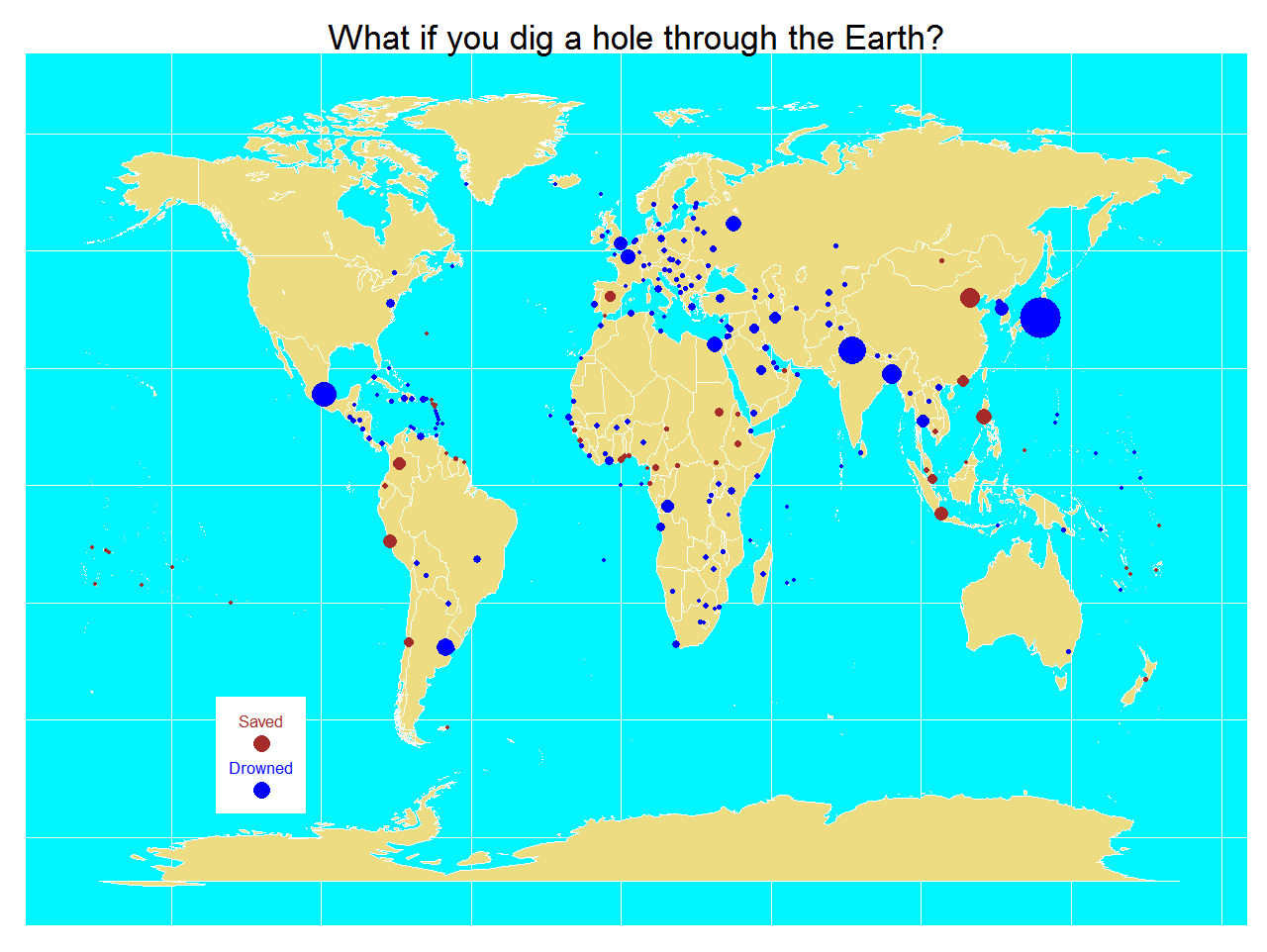By the way, I am one of the 23% of lucky people that would find land in the other side. I live in Madrid, Spain:An if some rainy afternoon having little to do I dig a hole through the Earth, I will appear in a place called Weber, in New Zealand: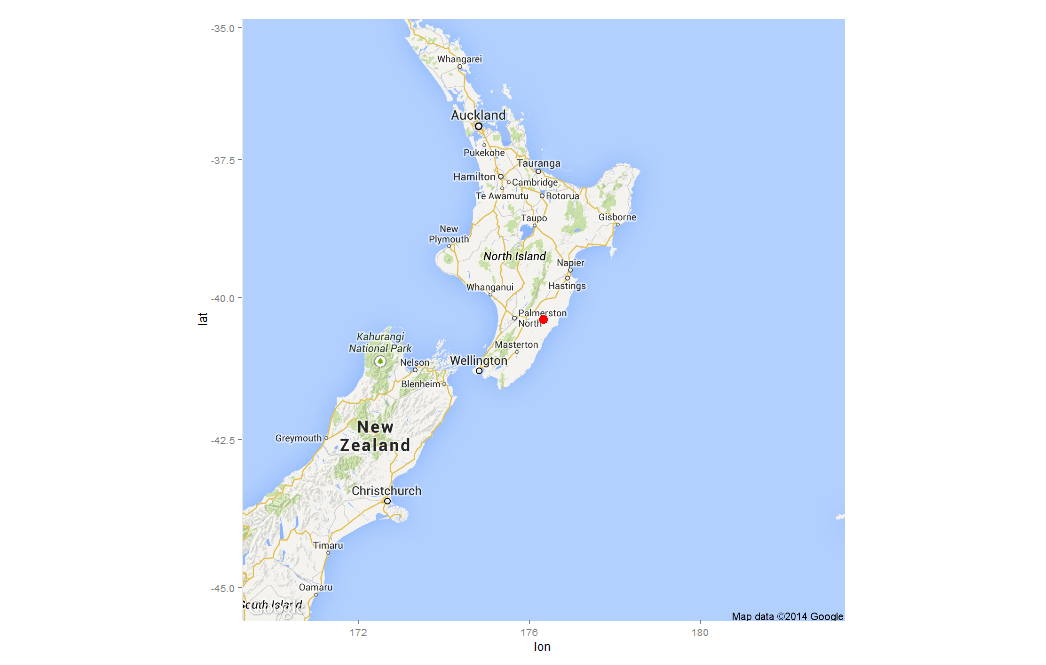This estimation can be very silly but the physics involved in the experiment are very interesting as you can see here. By the way, there is a film called Total Recall (2012) where the only way to travel between last two cities in the world is using an elevator through the Earth. Here you have the code:

```library(xlsx)
library(ggmap)
library(mapdata)
library(ggplot2)
#The xls file is in http://esa.un.org/unpd/wup/CD-ROM/WUP2011-F13-Capital_Cities.xls
names(CapitalCities) = gsub("\\.", "", names(CapitalCities))
#Obtain symmetric coordinates for each capital
CapitalCities\$LatitudeSym <- -CapitalCities\$Latitude
CapitalCities\$LongitudeSym <- -sign(CapitalCities\$Longitude)*(180-abs(CapitalCities\$Longitude))
CapitalCities\$DigResult <- apply(CapitalCities, 1, function(x) {unlist(revgeocode(c(as.numeric(x),as.numeric(x))))})
CapitalCities\$Drowned <- is.na(CapitalCities\$DigResult)*1
#Percentage of population saved
sum(CapitalCities\$Drowned*CapitalCities\$Populationthousands)/sum(CapitalCities\$Populationthousands)
world <- map_data("world")
opt <- theme(legend.position="none",
axis.ticks=element_blank(),
axis.title=element_blank(),
axis.text =element_blank(),
plot.title = element_text(size = 35),
panel.background = element_rect(fill="turquoise1"))
p <- ggplot()
p <- p + geom_polygon(data=world, aes(x=long, y=lat, group = group),colour="white", fill="lightgoldenrod2" )
p <- p + geom_point(data=CapitalCities, aes(x=Longitude, y=Latitude, color=Drowned, size = Populationthousands)) + scale_size(range = c(2, 20), name="Population (thousands)")
p <- p + labs(title = "What if you dig a hole through the Earth?")
p <- p + scale_colour_gradient(low = "brown", high = "blue")
p <- p + annotate("rect", xmin = -135, xmax = -105, ymin = -70, ymax = -45, fill = "white")
p <- p + annotate("text", label = "Drowned", x = -120, y = -60, size = 6, colour = "blue")
p <- p + annotate("text", label = "Saved", x = -120, y = -50, size = 6, colour = "brown")
p <- p + geom_point(aes(x = -120, y = -65), size=8, colour="blue")
p <- p + geom_point(aes(x = -120, y = -55), size=8, colour = "brown")
p + opt
# Get a map of Spain, centered and signed in Madrid
map.madrid <- get_map( location = as.numeric(madrid), color = "color", maptype = "roadmap", scale = 2, zoom = 6)
ggmap(map.madrid) + geom_point(aes(x = lon, y = lat), data = madrid, colour = 'red', size = 4)
# Get a map of New Zealand, centered and signed in Weber (the antipode of Madrid)
weber <- geocode('Weber, New Zealand')
map.weber <- get_map( location = as.numeric(weber), color = "color", maptype = "roadmap", scale = 2, zoom = 6)
ggmap(map.weber) + geom_point(aes(x = lon, y = lat), data = weber, colour = 'red', size = 4)
```

(1) United Nations, Department of Economic and Social Affairs, Population Division (2012). World Urbanization Prospects: The 2011 Revision, CD-ROM Edition.

# Do Not Play With Mr. Penney

Facts do not speak (Henry Poincare)

Mr. Penney is my best friend. He is maths teacher and loves playing. Yesterday we were in his office at the university when he suggested me a game:

When you toss a coin three times, you can obtain eight different sequences of tails and heads: TTT, TTH, THT, HTT, THH, HTH, HHT and HHH. Using a fair coin, all sequences have the same chances to appear. Choose one sequence and I will then choose another one. I will toss a coin until either your or my sequence appears as a consecutive subsequence of the coin toss outcomes. The player whose sequence appears first wins. I will repeat this procedure 100 times. The one with more games won is the winner of the game.  Don’t worry: I will not toss the coin manually. I will simulate using my computer. What’s your bet?

Ok, my bet is THT, I said. After some seconds, Mr. Penney said: My bet is TTH.

This was the result of the first round:Another chance? told me Mr. Penney. Of course! Now my bet is TTH! I said. In fact, I was thinking Take that! Now I chose your previous bet. Do you think I am foolish?. After some seconds, Mr. Penney said: My bet now is HTT.

This was the result of the second round:Another chance? told me Mr. Penney. At this point, I was very suspicious but I wanted the last chance so I told him Of course! Now my bet is HTT! I wanted to try my strategy one more time. After some seconds, Mr. Penney said: My bet now is HHT.

This was the result of the third round:Ok, I give it up! What’s the trick? I said. And Mr. Penney explained it to me. You can find the explanation here. This is the last time I play with you! I told him once he finished the explanation.

Here you have the code. Feel free to play:

```library(gridExtra)
library(gridExtra)
Me     <- "TTH"
Penney <- "HTT"
results <- data.frame(play= numeric(0), Penney = integer(0), Me = character(0))
for (i in 1:100) {
play <- c()
repeat {play <- do.call(paste, c(play, as.list(sample(c("H","T"), 1)), sep=""))
if (grepl(Penney, play)|grepl(Me, play)) {
results <- rbind(results, data.frame(play= i, Penney = as.numeric(grepl(Penney, play)), Me = as.numeric(grepl(Me, play))))
break}}}
grid.newpage()
table <- rbind(
c("Me", Me, sum(results\$Me), if(sum(results\$Penney) > sum(results\$Me)) "Loser" else "Winner"),
c("Penney", Penney, sum(results\$Penney), if(sum(results\$Penney) > sum(results\$Me)) "Winner" else "Loser"))
grid.table(table,
cols = c("Player", "Bet", "Games Won", "Result"),
gpar.colfill = gpar(fill="palegreen3",col="White"),
gpar.corefill =  gpar(fill="palegreen",col="White"),
gpar.rowfill = gpar(fill=NA, col=NA))
```

# Random Love

Anyone who considers arithmetical methods of producing random digits is, of course, in a state of sin (John von Newman)

Ms. Positive and Mr. Negative live in a one-dimensional world and are falling in love. But beginnings are not always easy. They have a big problem: none of them like the other’s neighborhood. Ms. Positive only wants to walk around Positive Integer Numbers Neighborhood and Mr. Negative around Negative Integers Numbers one. This is a prickly problem they need to deal with as soon as possible. But they have a good idea. They will start their walks from Zero, an impartial place between both neighborhoods and will let fate to guide their feet. They will toss a coin to decide every step: if result is head, they will advance 1 step toward positive numbers neighborhood; if tail, they will advance 1 step toward negative numbers one. For example, if the first 5 tosses are face, face, tail, tail and tail, the their first 5 steps will be +1, +2, +1, 0 and -1. It seems to be a fair agreement for both. Maybe is not the most pleasant way to take a walk but It is well known that lovers use to do silly things constantly, especially at the beginnings. They always walk for two hours, so they toss the coin 7.200 times every walk (these lovers are absolutely crazy as you can see). This was their first walk:After this first walk, Mr Negative was really upset. Ms. Positive, watching his face fell, ask him: What’s the matter, honey? and Mr. Negative replied: What’s the matter? What’s the matter? The matter is that we spent almost all the time walking around your horrible neighborhood! What comes next is too hard to be reproduced here. Anyway, they agreed to give a chance to the method they designed. How can one imagine that a coin can produce such a strange walk! There must be an error! After 90 walks, the situation of our lovers was extremely delicate. A 57% of the walks were absolutely awful for one of them since more than 80% of the steps were around the same neighborhood. Another 32% were a bit uncomfortable for one of them since between 60% and 80% of the steps were around the same neighborhood. Only 11% of the walks were gratifying. How is it possible?, said Mr. Negative. How is it possible?, said Ms. Positive.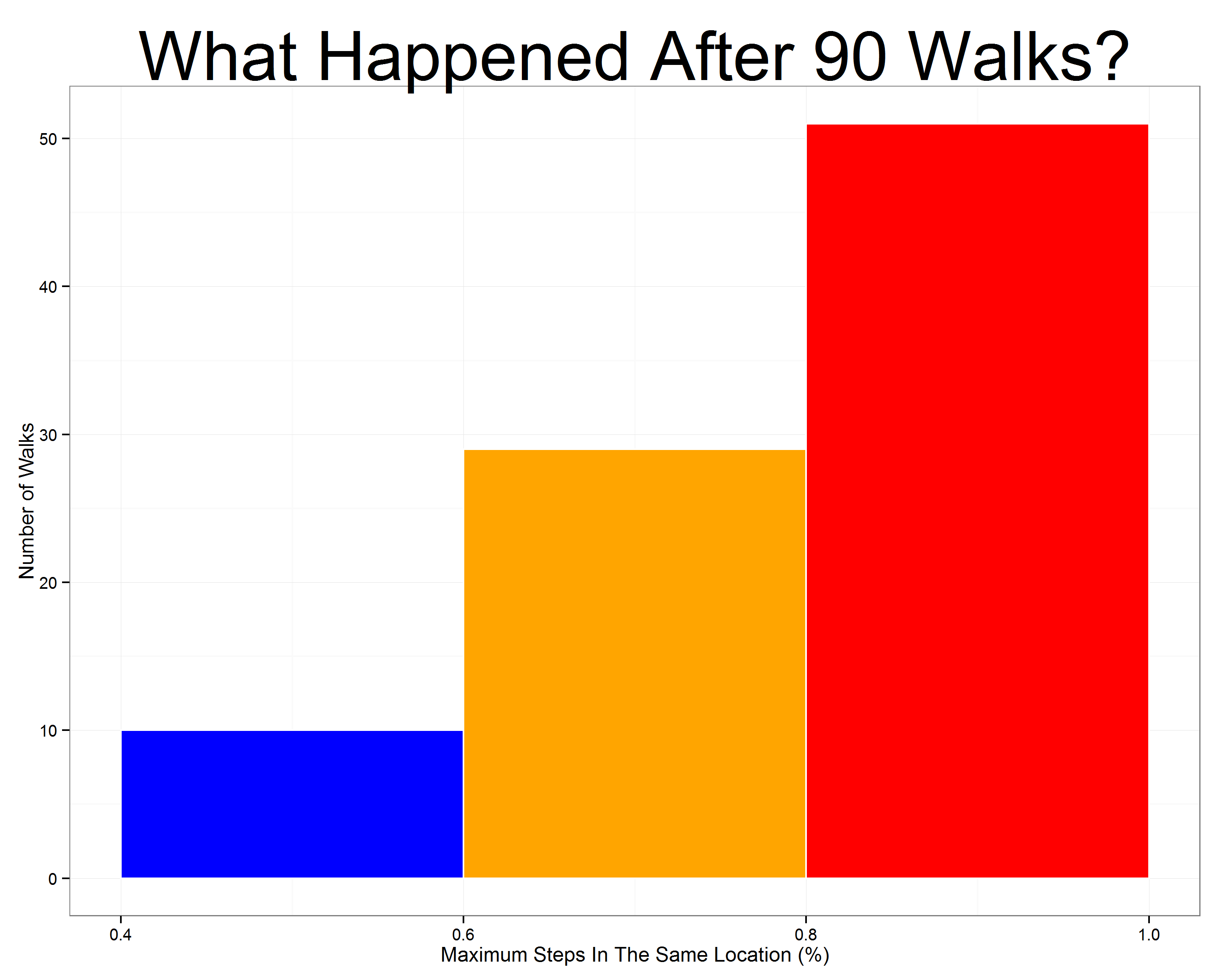But here comes Ms. Positive, who always looks on the brigth side of life: Don’t worry, darling. In fact, we don’t have to be sad. We get angry the same amount of times! For me is enough. What about you?, said her. For me is perfect as well!, said Mr. Negative. In that moment, they realise they were made for each other and started another random walk with a big smile on their faces.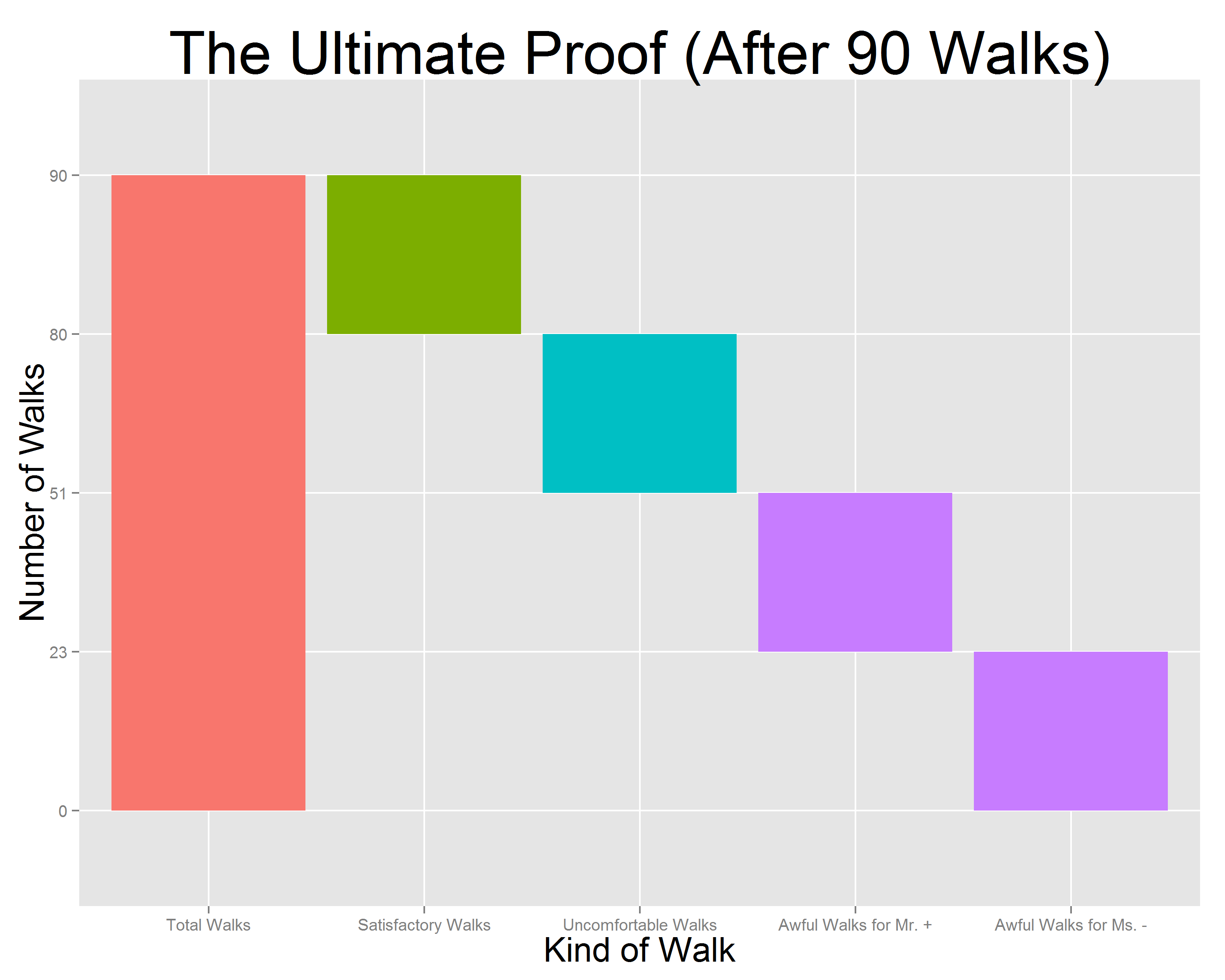This is the code:

```library(ggplot2)
steps   <- 2*60*60 #Number of steps
results <- data.frame()
walks<-90 #Number of walks
for (i in 1:walks)
{
state <- cumsum(sample(c(-1,1), steps, replace = TRUE))
results <- rbind(results, c(sum(state<0), sum(state>0), sum(state==0),
if (sum(state<0) >= sum(state>0)) 1 else 0))
}
colnames(results) <- c("neg.steps", "pos.steps", "zero.steps", "ind.neg")
results\$max.steps <- apply(results, 1, max)/apply(results, 1, sum)
#Plot of one of these walks
mfar=max(abs(max(state)),abs(min(state)))
plot1 <- qplot(seq_along(state),
state,
geom="path")+
xlab("Step") +
ylab("Location") +
labs(title = "The First Walk Of Ms. Positive And Mr. Negative")+
theme(plot.title = element_text(size = 35))+
theme(axis.title.y = element_text(size = 20))+
theme(axis.title.x = element_text(size = 20))+
scale_x_continuous(limits=c(0, length(state)),breaks=c(1,steps/4,steps/2,3*steps/4,steps))+
scale_y_continuous(limits=c(-mfar, mfar), breaks=c(-mfar,-mfar/2, 0, mfar/2,mfar))+
geom_hline(yintercept=0)
ggsave(plot1, file="plot1.png", width = 12, height = 10)
#Summary of all walks
hist1 <- ggplot(results, aes(x = max.steps))+
geom_histogram(colour = "white",breaks=seq(.4,1,by=.2),fill=c("blue", "orange", "red"))+
theme_bw()+
labs(title = paste("What Happened After ", toString(walks), " Walks?",sep = ""))+
scale_y_continuous(breaks=seq(0,(nrow(results[results\$max.steps>.8,])+10),by=10))+
theme(plot.title = element_text(size = 40))+
xlab("Maximum Steps In The Same Location (%)") +
ylab("Number of Walks")
ggsave(hist1, file="hist1.png", width = 10, height = 8)
#Data for waterfall chart
waterfall <- as.data.frame(cbind(
c("Total Walks", "Satisfactory Walks", "Uncomfortable Walks", "Awful Walks for Mr. +", "Awful Walks for Ms. -"),
c("a", "b", "c", "d", "d"),
c(0,
nrow(results),
nrow(results)-nrow(results[results\$max.steps<.6,]),
nrow(results)-nrow(results[results\$max.steps<.6,])-nrow(results[results\$max.steps>=.6 & results\$max.steps<.8,]),
nrow(results)-nrow(results[results\$max.steps<.6,])-nrow(results[results\$max.steps>=.6 & results\$max.steps<.8,])-nrow(results[results\$max.steps>=.8 & results\$ind.neg==1,])
),
c(nrow(results),
nrow(results)-nrow(results[results\$max.steps<.6,]),
nrow(results)-nrow(results[results\$max.steps<.6,])-nrow(results[results\$max.steps>=.6 & results\$max.steps<.8,]),
nrow(results)-nrow(results[results\$max.steps<.6,])-nrow(results[results\$max.steps>=.6 & results\$max.steps<.8,])-nrow(results[results\$max.steps>=.8 & results\$ind.neg==1,]),
0
),
c(nrow(results),
nrow(results[results\$max.steps<.6,]),
nrow(results[results\$max.steps>=.6 & results\$max.steps<.8,]),
nrow(results[results\$max.steps>=.8 & results\$ind.neg==1,]),
nrow(results[results\$max.steps>=.8 & results\$ind.neg==0,]))
))
colnames(waterfall) <-c("desc", "type", "start", "end", "amount")
waterfall\$id <- seq_along(waterfall\$amount)
waterfall\$desc <- factor(waterfall\$desc, levels = waterfall\$desc)
#Waterfall chart
water1 <- ggplot(waterfall, aes(desc, fill = type)) +
geom_rect(aes(x = desc, xmin = id-0.45, xmax = id+0.45, ymin = end, ymax = start))+
xlab("Kind of Walk") +
ylab("Number of Walks") +
labs(title = "The Ultimate Proof (After 90 Walks)")+
theme(plot.title = element_text(size = 35))+
theme(axis.title.y = element_text(size = 20))+
theme(axis.title.x = element_text(size = 20))+
theme(legend.position = "none")
```

# Dora’s Choice

Arithmetic is being able to count up to twenty without taking off your shoes (Mickey Mouse)On her last mission, Dora The Explorer sails down the Amazon river to save her friend Isa The Iguana from Swiper The Fox claws. After some hours of navigation, Dora sees how the river divides into 3 branches and has to choose which one to follow. Before leaving, her friend Map told her that just one of these branches is safe. Two others end in terrible waterfalls, both impossible to escape alive. Although Dora does not know which one is the good one, she decides to take the branch number 1. Suddenly, her friend Boots The Monkey yells from the top of a palm tree:

Dora, do not take branch number 3! I can see from here that it ends in a horrible waterfall!

After listening to Boots, Dora changes her mind and decides to take branch number 2. Why Dora switches? Because she knows that this change has significantly increased her probability of ending the mission alive.

There are several ways to convince yourself of this. One is to simulate the situation that has faced Dora and compare results of switching and not switching . Switching, Dora saves her life 2 of each 3 simulations while if she does not, Dora only saves 1 of each 3 simulations. Changing her mind, Dora doubles her chances of survival!

Carefully considering what happens, you can see that switching Dora saves herself when her first choice is erroneus, which occurs with probability 2/3. On the other hand, if Dora remains faithful to her first choice, obviously only saves herself with probability 1/3.

This is an example on my own of the famous Monty Hall Problem. You can see a nice explanation of it in a chapter of Numb3rs or in the film 21 Black Jack. Not long ago I exposed the problem in a family meeting. Only my mum said she would switch (we were 6 people in the meeting). It is fun to share this experiment and ask what people would do. Do it with your friends and family. First time I knew the problem I thought there were no difference between switching and not since I gave both possibilities 1/2 of probability. If I had been Dora, pretty sure I would tumbled over a terrible waterfall. What about yo?

Note: this is an update of the post, which was not a correct formulation of Monty Hall Problem. Thanks to David Robinson and Scott Kostyshak for showing me my error. A correct formulation of the problem may be this:

On her last mission, Dora The Explorer sails down the Amazon river to meet her cousin Diego. After some hours of navigation, Dora sees how the river divides into 3 branches and has to choose which one to follow. Before leaving, her friend Map told her that just one of these branches is safe. Two others end in terrible waterfalls, both impossible to escape alive. Although Dora does not know which one is the good one, she decides to take the branch number 1. After putting the bow towards branch number one, Dora sees Swiper The Fox smiling from the shore, in a high place where obviously can see the end of all three branches. Dora yells him:

– Help me Swiper! Which one should I take?

Swiper replies:

– I am the villain of this story so I will give you only an advice: do not take branch number 3. It ends into a terrible waterfall.

Dora, who has a sixth sense to notice when Swiper is lying, knows he is telling the truth and immediately changes her mind and decides to take branch number 2. Why Dora switches? Because she knows that this change has significantly increased her probability of ending the mission alive.Here you have the code:

```library(ggplot2)
library(extrafont)
nchoices <- 3
nsims <- 500
choices <- seq(from=1, to=nchoices, by=1)
good.choice <- sample(choices, nsims, replace=TRUE)
choice1 <- sample(choices, nsims, replace=TRUE)
dfsims <- as.data.frame(cbind(good.choice, choice1))
dfsims\$advice <- apply(dfsims, 1, function(x) choices[!choices %in% as.vector(x)][sample(1:length(choices[!choices %in% as.vector(x)]), 1)])
dfsims\$choice2 <- apply(dfsims, 1, function(x) choices[!choices %in% as.vector(c(x, x))][sample(1:length(choices[!choices %in% as.vector(c(x, x))]), 1)])
dfsims\$win1 <- apply(dfsims, 1, function(x) (x==x)*1)
dfsims\$win2 <- apply(dfsims, 1, function(x) (x==x)*1)
dfsims\$csumwin1 <- cumsum(dfsims\$win1)/as.numeric(rownames(dfsims))
dfsims\$csumwin2 <- cumsum(dfsims\$win2)/as.numeric(rownames(dfsims))
dfsims\$nsims <- as.numeric(rownames(dfsims))
dfsims\$xaxis <- 0
### XKCD theme
theme_xkcd <- theme(
panel.background = element_rect(fill="darkolivegreen1"),
panel.border = element_rect(colour="black", fill=NA),
axis.line = element_line(size = 0.5, colour = "black"),
axis.ticks = element_line(colour="black"),
panel.grid = element_line(colour="white", linetype = 2),
axis.text.y = element_text(colour="black"),
axis.text.x = element_text(colour="black"),
text = element_text(size=18, family="Humor Sans"),
plot.title = element_text(size = 50)
)
### Plot the chart
p <- ggplot(data=dfsims, aes(x=nsims, y=csumwin1))+
geom_line(aes(y=csumwin2), colour="green4", size=1.5, fill=NA)+
geom_line(colour="green4", size=1.5, fill=NA)+
geom_text(data=dfsims[400, ], family="Humor Sans", aes(x=nsims), colour="green4", y=0.7, label="if Dora switches ...", size=5.5, adjust=1)+
geom_text(data=dfsims[400, ], family="Humor Sans", aes(x=nsims), colour="green4", y=0.3, label="if Dora does not switch ...", size=5.5, adjust=1)+
coord_cartesian(ylim=c(0, 1), xlim=c(1, nsims))+
scale_y_continuous(breaks = c(0,round(1/3, digits = 2),round(2/3, digits = 2),1), minor_breaks = c(round(1/3, digits = 2),round(2/3, digits = 2)))+
scale_x_continuous(minor_breaks = seq(100, 400, 100))+
labs(x="Number Of Simulations", y="Rate Of Survival", title="Dora's Choice")+
theme_xkcd
ggsave("doras_choice.jpg", plot=p, width=8, height=5)
```

# What The Hell Is Pi Doing Here?

Nothing in Nature is random … A thing appears random only through the incompleteness of our knowledge (Benedict Spinoza)

This is one of my favorite mathematical mysteries. In 1991 David Boll was trying to confirm that the neck of the Mandelbrot Set is 0 in thickness. Neck is located at -0.75+0i (where two biggest circles meet each other).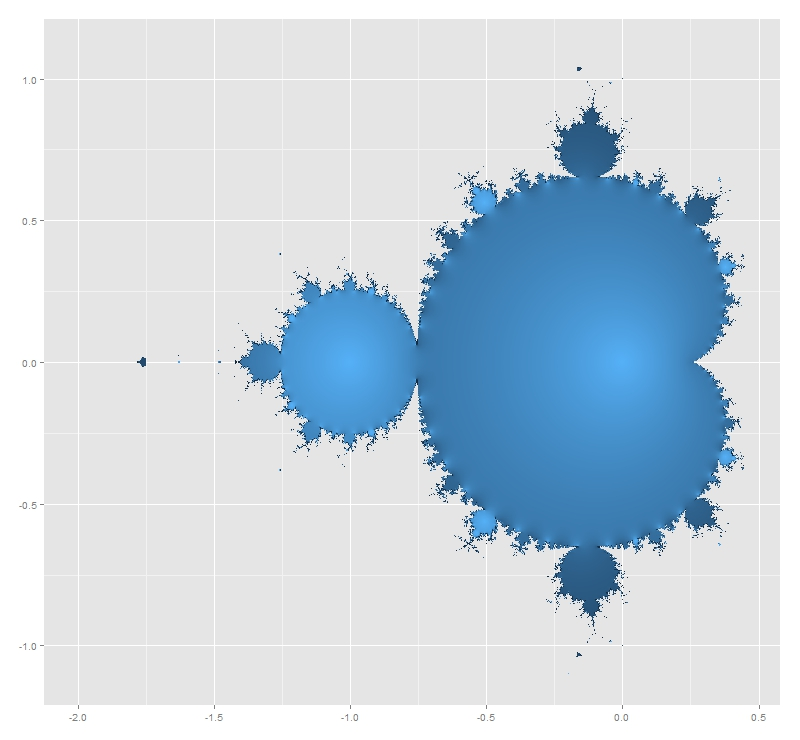He tried with complex numbers like -0.75+εi for small values of ε demonstrating the divergence of all these numbers. And here comes the mystery: multiplying ε and the corresponding number of iterations it took for the iterate to diverge, gives an approximation of π that is within ±ε. Is not fascinating? I replicated David Boll’s experiment for positive and negative values of ε. I draw results as follows: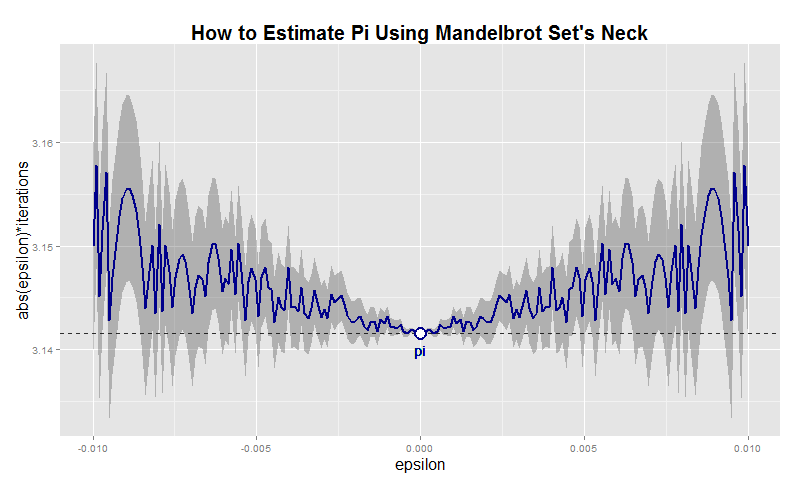Before doing it, I thought I was going to find some pattern in the graphic. Apart from the mirror effect produced by the sign of ε, there is nothing recognizable. Convergence is chaotic. Here you have the code. This example is also nice to practice with ggplot2 package, one of the totems of R:

```i<-0    # Counter of iterations
x  <- 0 # Initialization
while (Mod(x) <= 2)
{
x <- x^2+(c+complex(real = 0, imaginary = e))
i <- i+1
}
i
}
results <- as.data.frame(c(NULL,NULL))
for (j in 1:length(epsilons))
{results <- rbind(results, c(epsilons[j], testMSConvergence(epsilons[j])))}
colnames(results) <- c('epsilon', 'iterations')
dev.off()
p <- ggplot(results, aes(epsilon,abs(epsilon)*iterations))+
xlab("epsilon")+
ylab("abs(epsilon)*iterations")+
opts(axis.title.x=theme_text(size=16)) +
opts(axis.title.y=theme_text(size=16)) +
ggtitle("How to Estimate Pi Using Mandelbrot Set's Neck")+
theme(plot.title = element_text(size=20, face="bold"))
p <- p + geom_ribbon(data=results,aes(ymin=abs(epsilon)*iterations-abs(epsilon),ymax=abs(epsilon)*iterations+abs(epsilon)), alpha=0.3)
p <- p + geom_abline(intercept = pi, , slope = 0, size = 0.4, linetype=2, colour = "black", alpha=0.8)
p <- p + geom_line(colour = "dark blue", size = 1, linetype = 1)
p <- p + geom_text(x = 0, y = pi, label="pi", vjust=2, colour="dark blue")
p <- p + geom_point(x = 0, y = pi, size = 6, colour="dark blue")
p + geom_point(x = 0, y = pi, size = 4, colour="white")
```